## Attack

### What do we want do？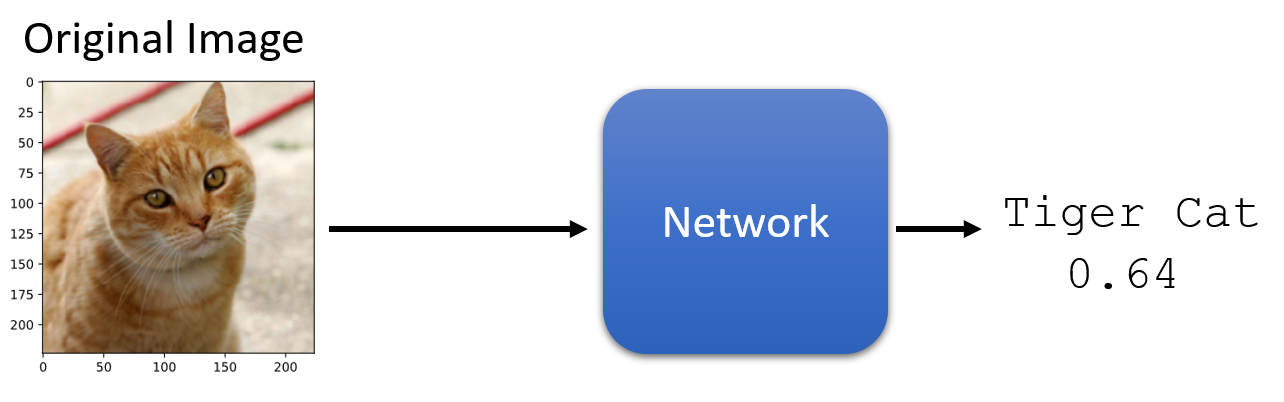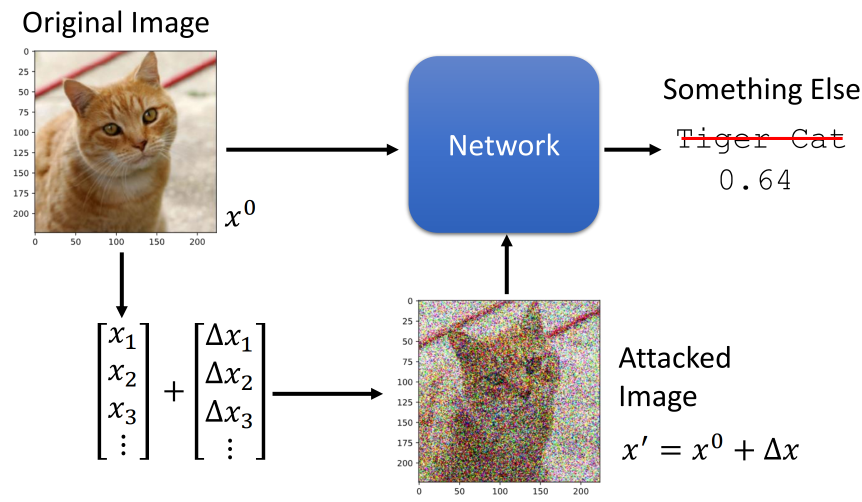### Loss function for Attack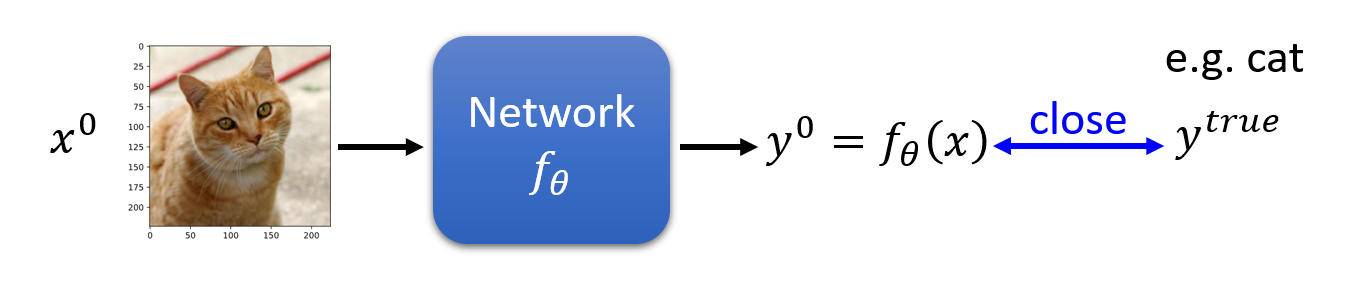$$Loss(\theta) = C(y^{0}, y^{true})$$

$$f^{*} = arg \min\limits_{\theta}Loss(\theta)$$

$$Loss(x^{‘}) = -C(y^{0}, y^{true})$$

$$Loss(x^{‘}) = -C(y^{0}, y^{true}) + C(y^{0}, y^{false})$$

$$d(X^{0}, X^{‘}) \leq \epsilon$$

### Constraint

$$d(X^{0}, X^{‘}) = {||X^{0} - X^{‘}||}_{2} = (\Delta x_1)^2 + (\Delta x_2)^2 + (\Delta x_3)^2+ …$$

$$d(X^{0}, X^{‘}) = {||X^{0} - X^{‘}||}_{\infty} = max { \Delta x_1, \Delta x_2, \Delta x_3， … }$$### How to attack?

$$X^{*} = arg\min\limits_{d(X^{0}, X^{‘}) \leq \epsilon} Loss(X^{‘})$$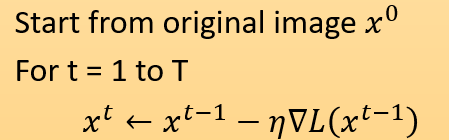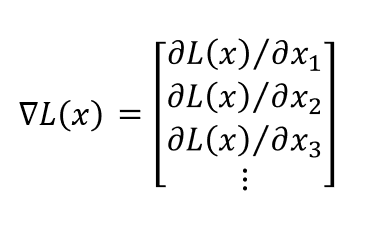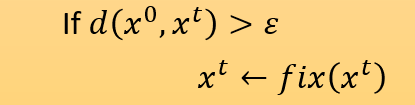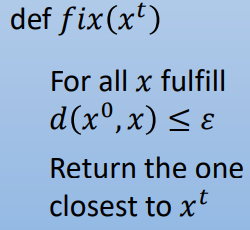$x^0 ​$表示图片是高维空间中的一点(向量)， 假设$x^0​$是二维向量，那么以$x^0​$为圆心， 以$\epsilon ​$为半径构成的圆，圆内所有的点都为满足条件的点。$x^t​$每更新一次都判断它在不在圆内，如果不在圆内$x^t​$就用满足条件且与$x^t​$距离最近的点替代。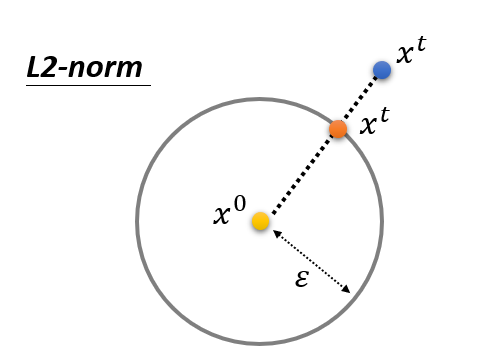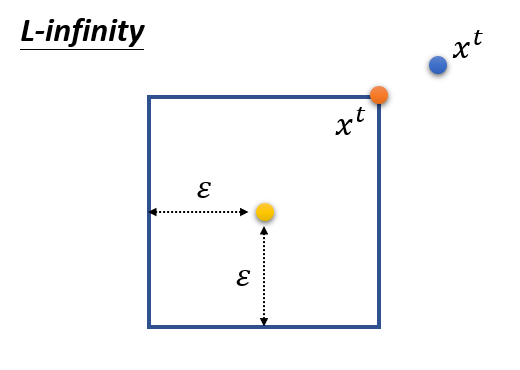### Example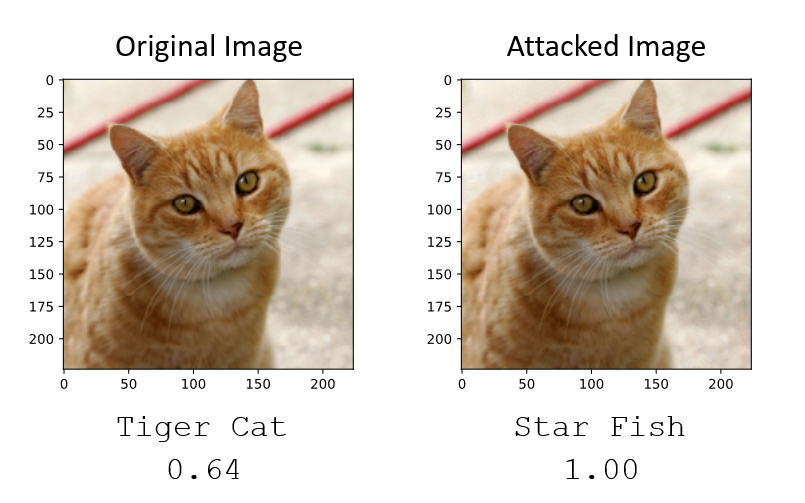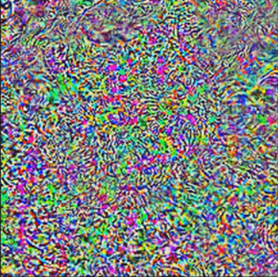### What Happened？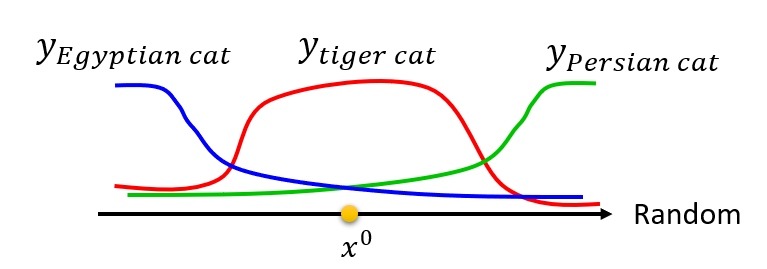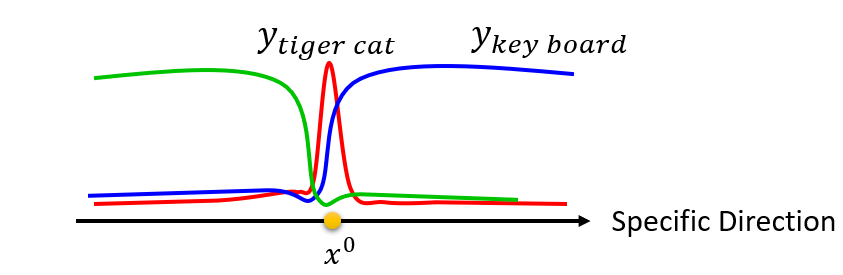### Attack Approaches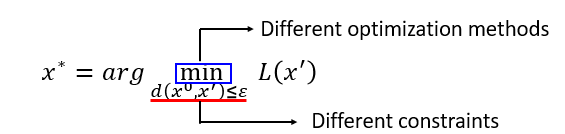• FGSM (https://arxiv.org/abs/1412.6572)

•Basic iterative method (https://arxiv.org/abs/1607.02533)

•L-BFGS (https://arxiv.org/abs/1312.6199)

•Deepfool (https://arxiv.org/abs/1511.04599)

•JSMA (https://arxiv.org/abs/1511.07528)

•Elastic net attack (https://arxiv.org/abs/1709.04114)

•Spatially Transformed (https://arxiv.org/abs/1801.02612)

•One Pixel Attack (https://arxiv.org/abs/1710.08864)

…..

### Fast Gradient Sign Method (FGSM)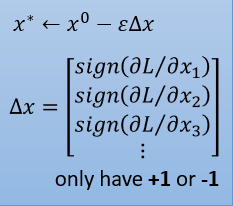FGSM只是把图片加上正负$\epsilon$，并且只Update一次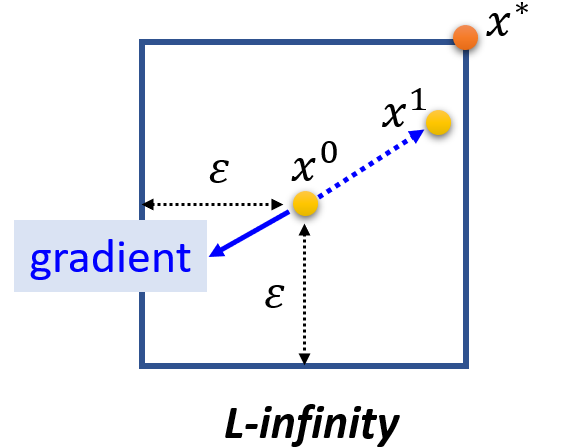### Black Box Attack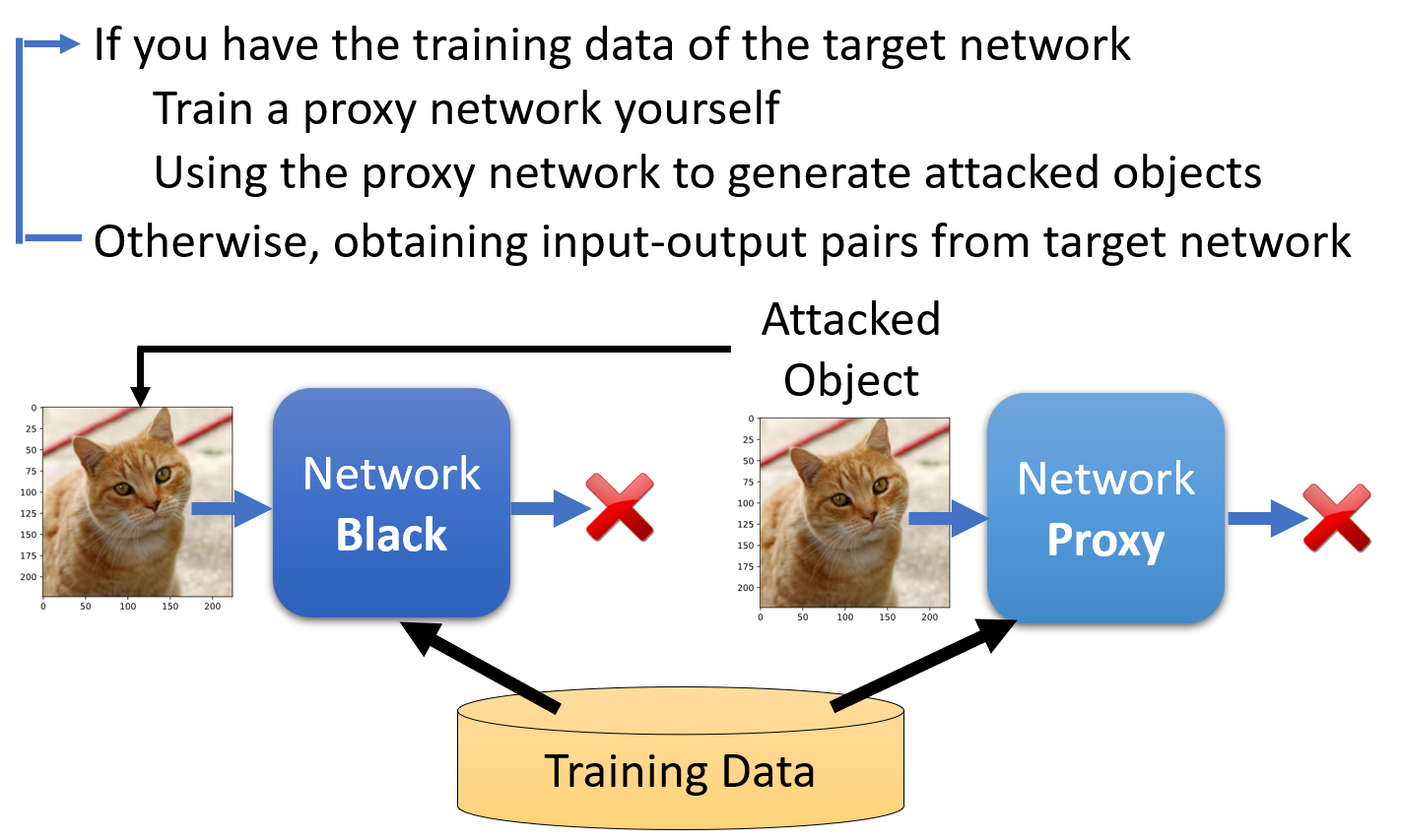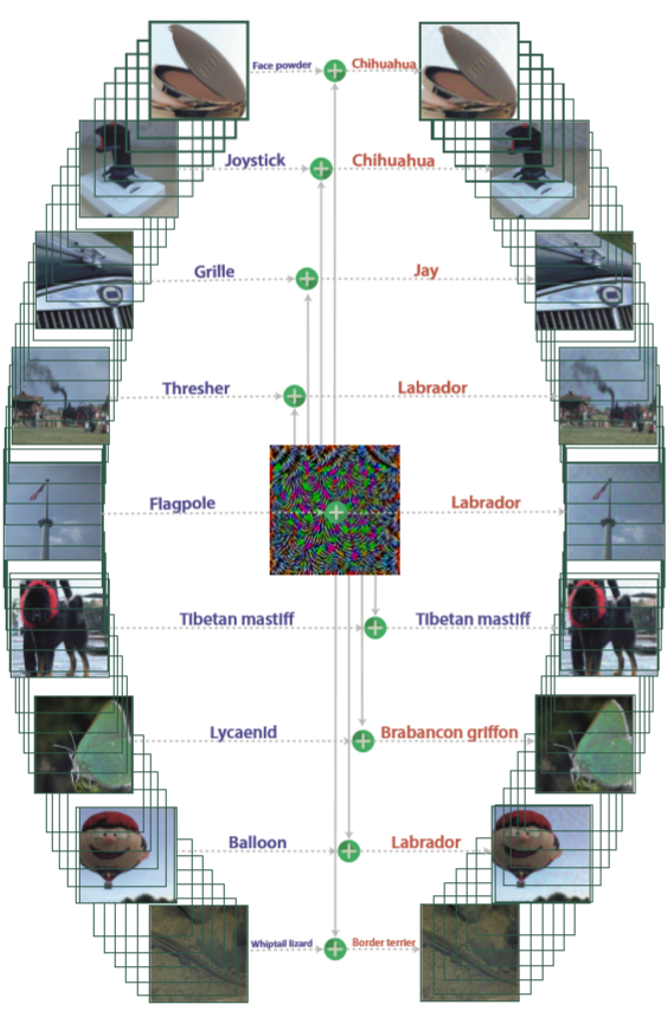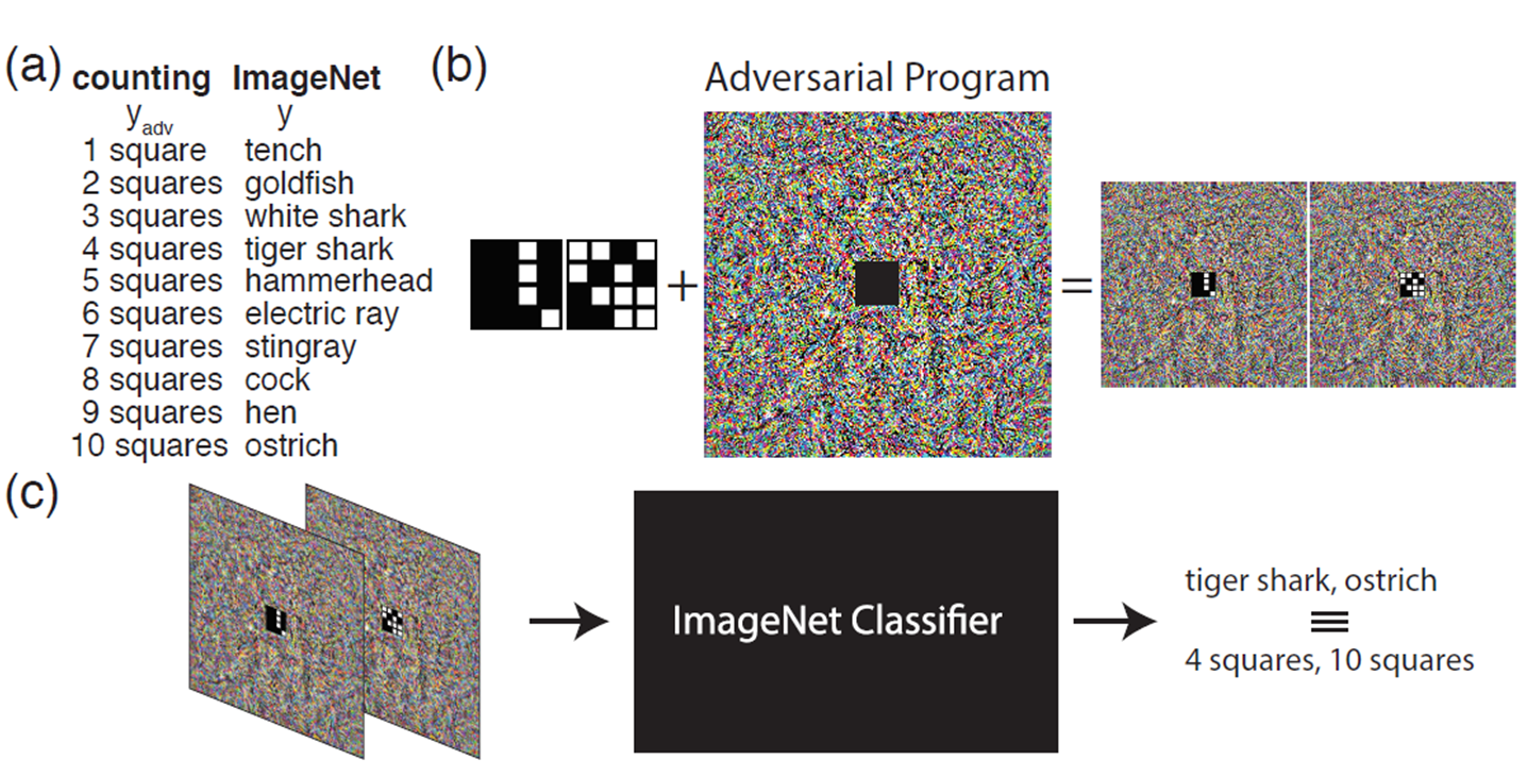### Attack in the Real World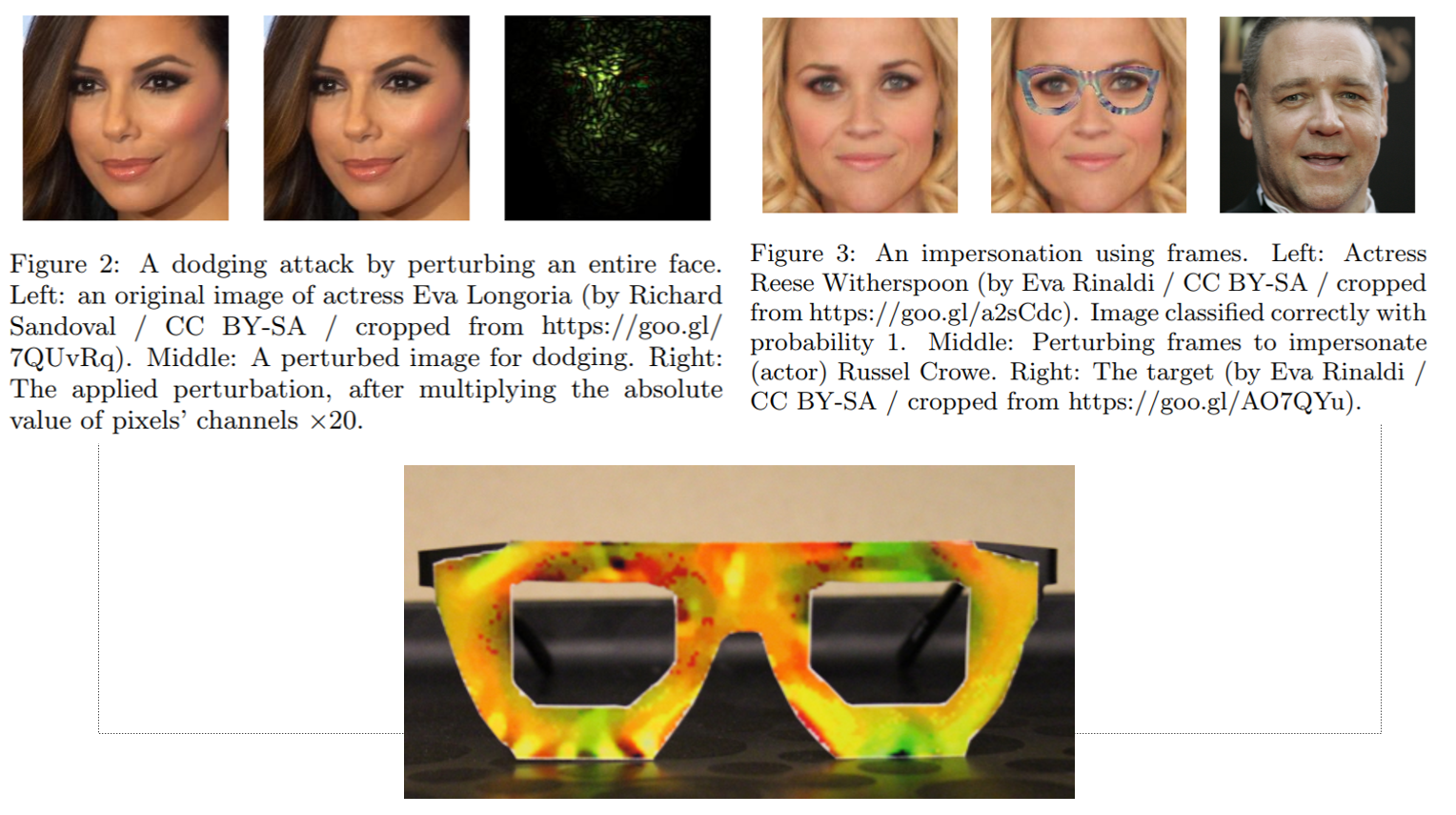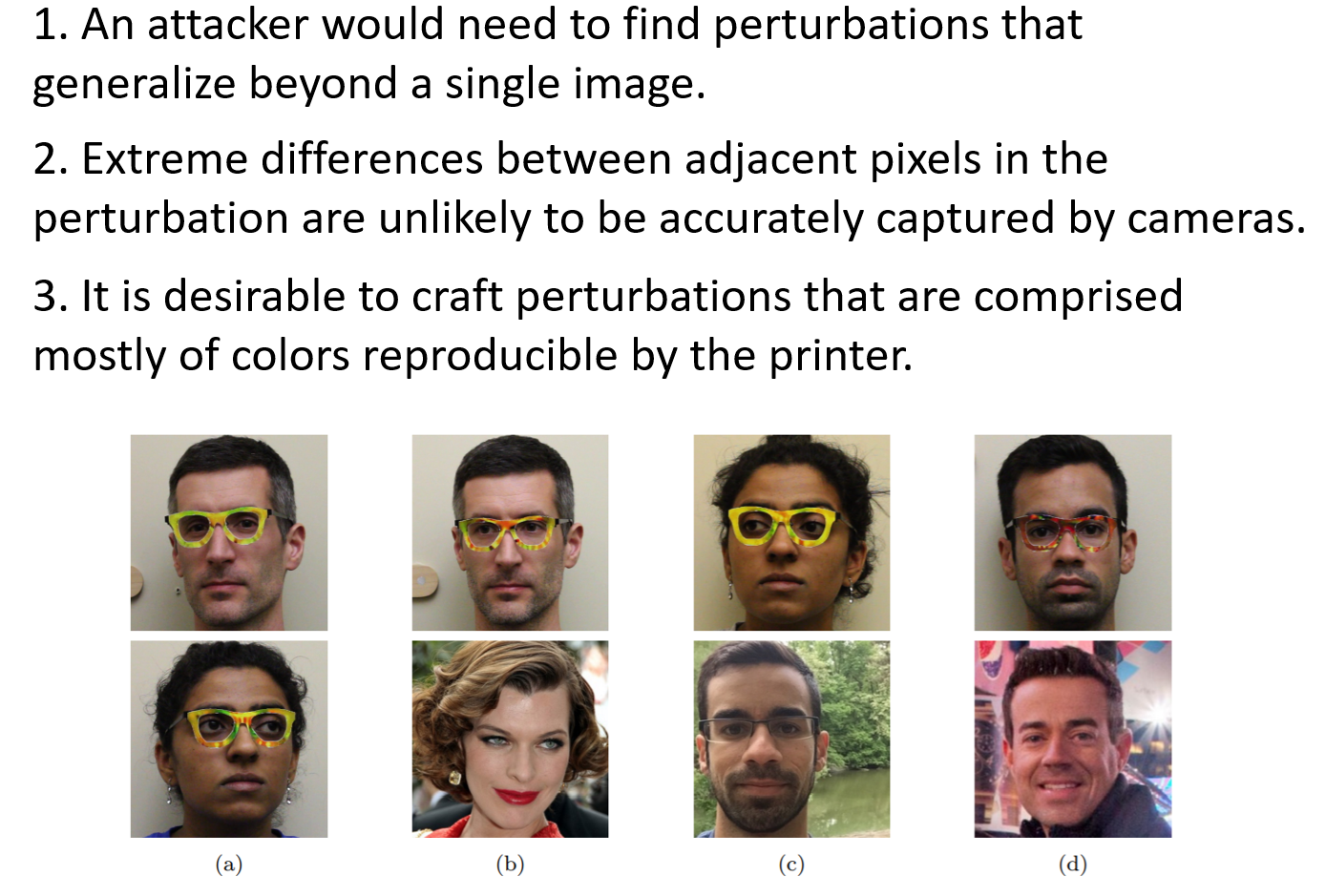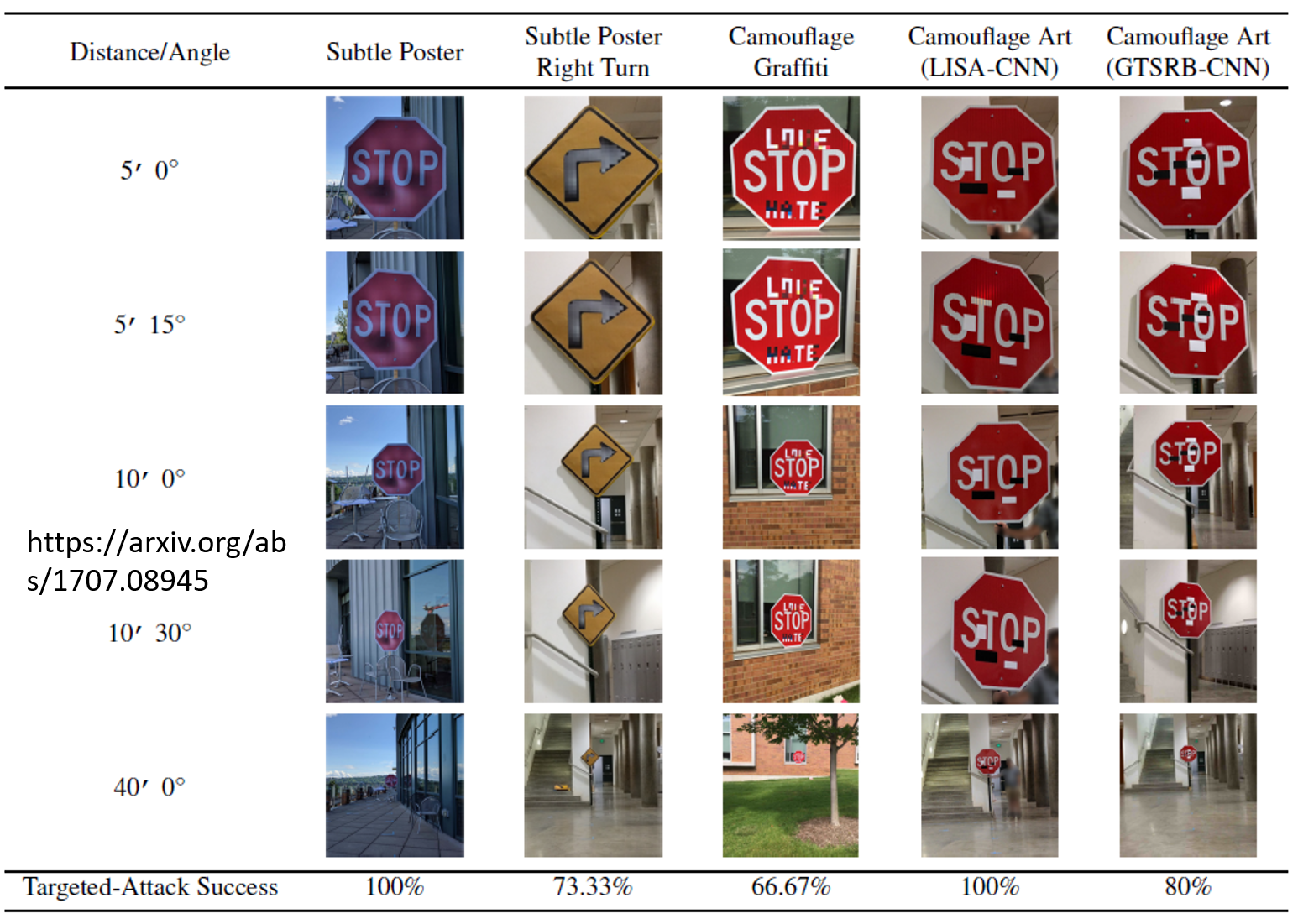## Defense

### Passive Defense

Passive Defense只是能够找出对抗样本，并不更改模型的参数，主体思想就是我们在把图片送到之前先把图片做一下处理(平滑化等)，这样可以减少噪音带来的影响。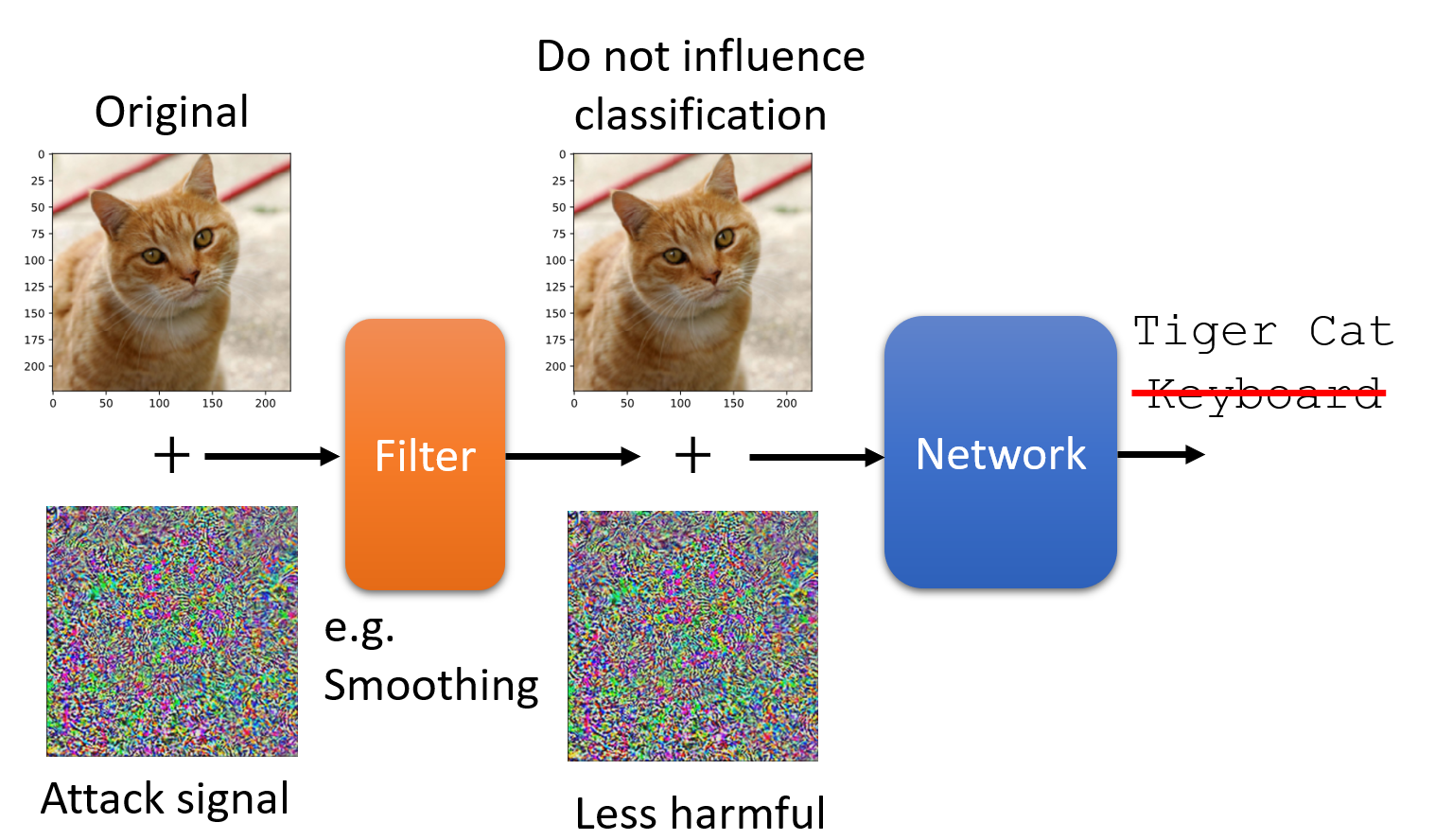#### Feature Squeeze

Squeezer指的就是前面提到的处理，如果三个预测结果差距很大就意味着图片受到攻击。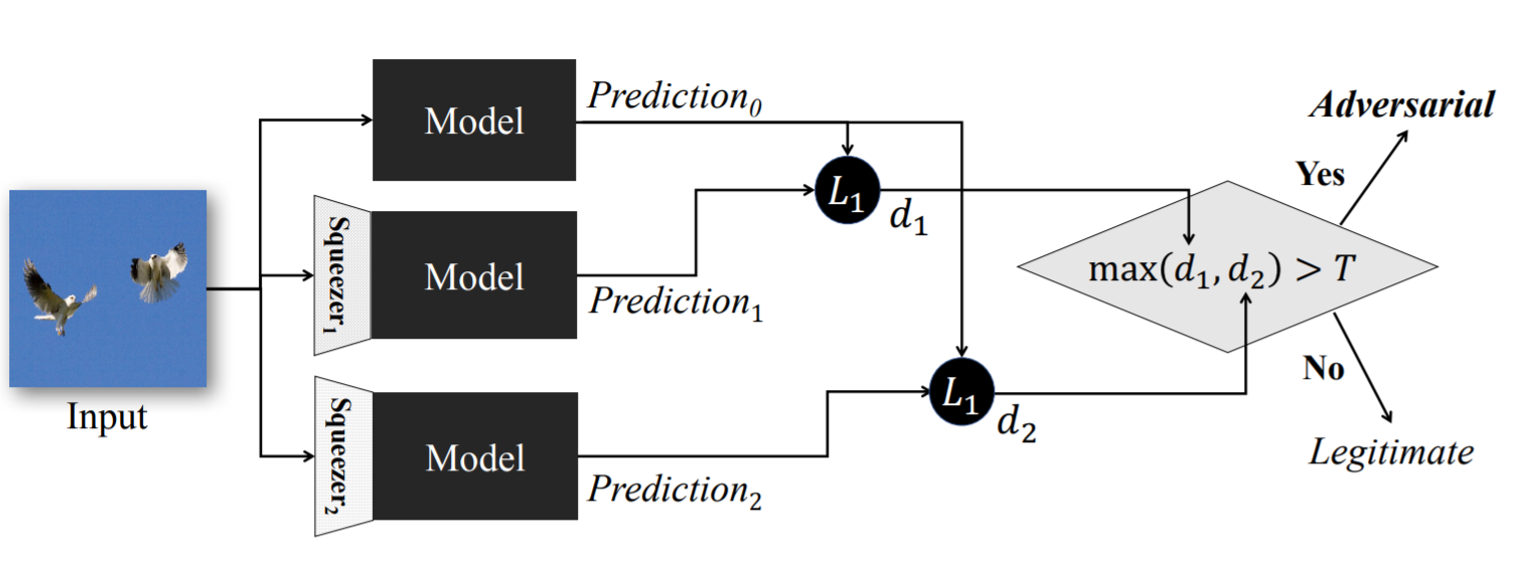#### Randomization at Inference Phase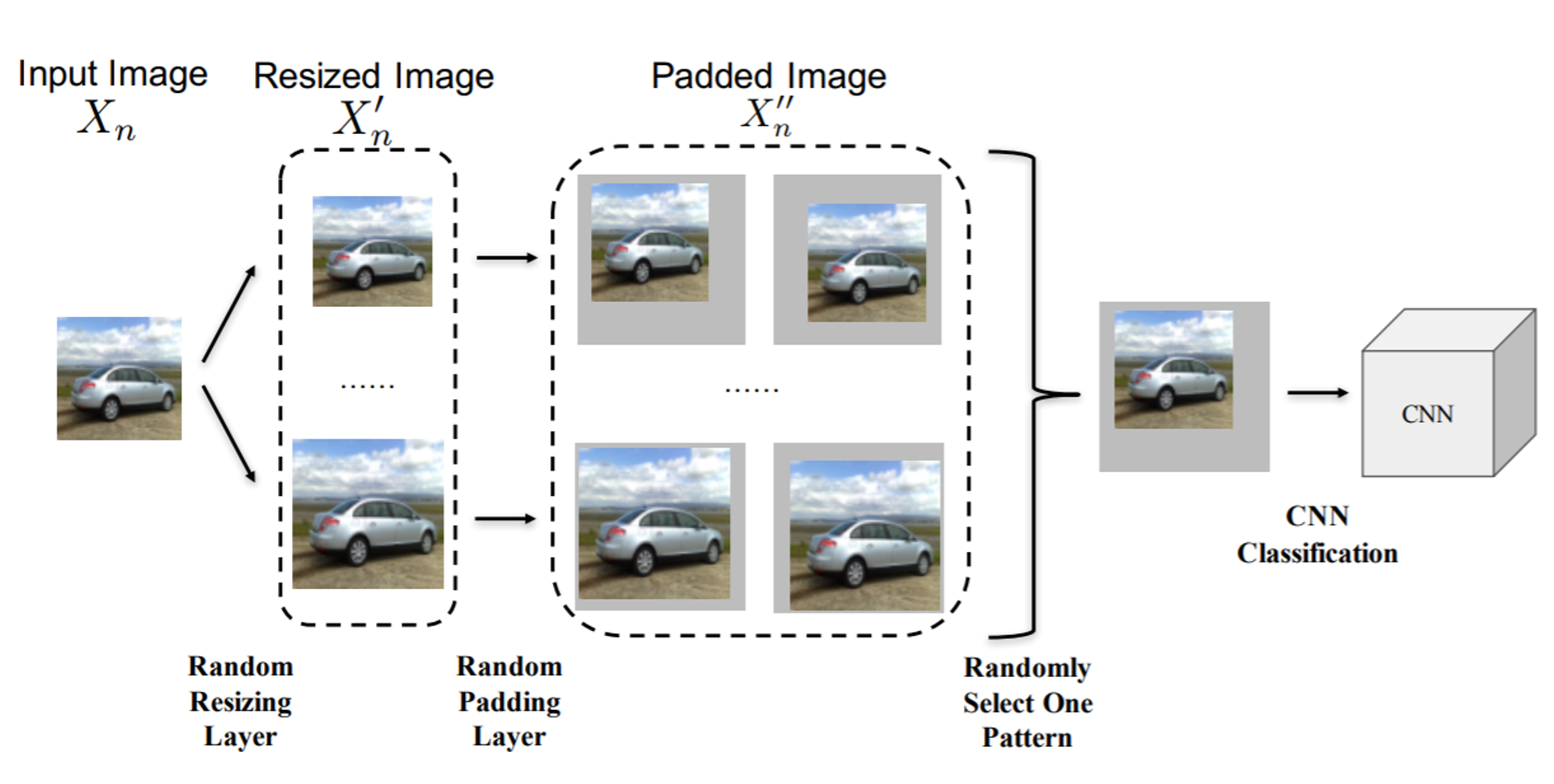### Proactive Defense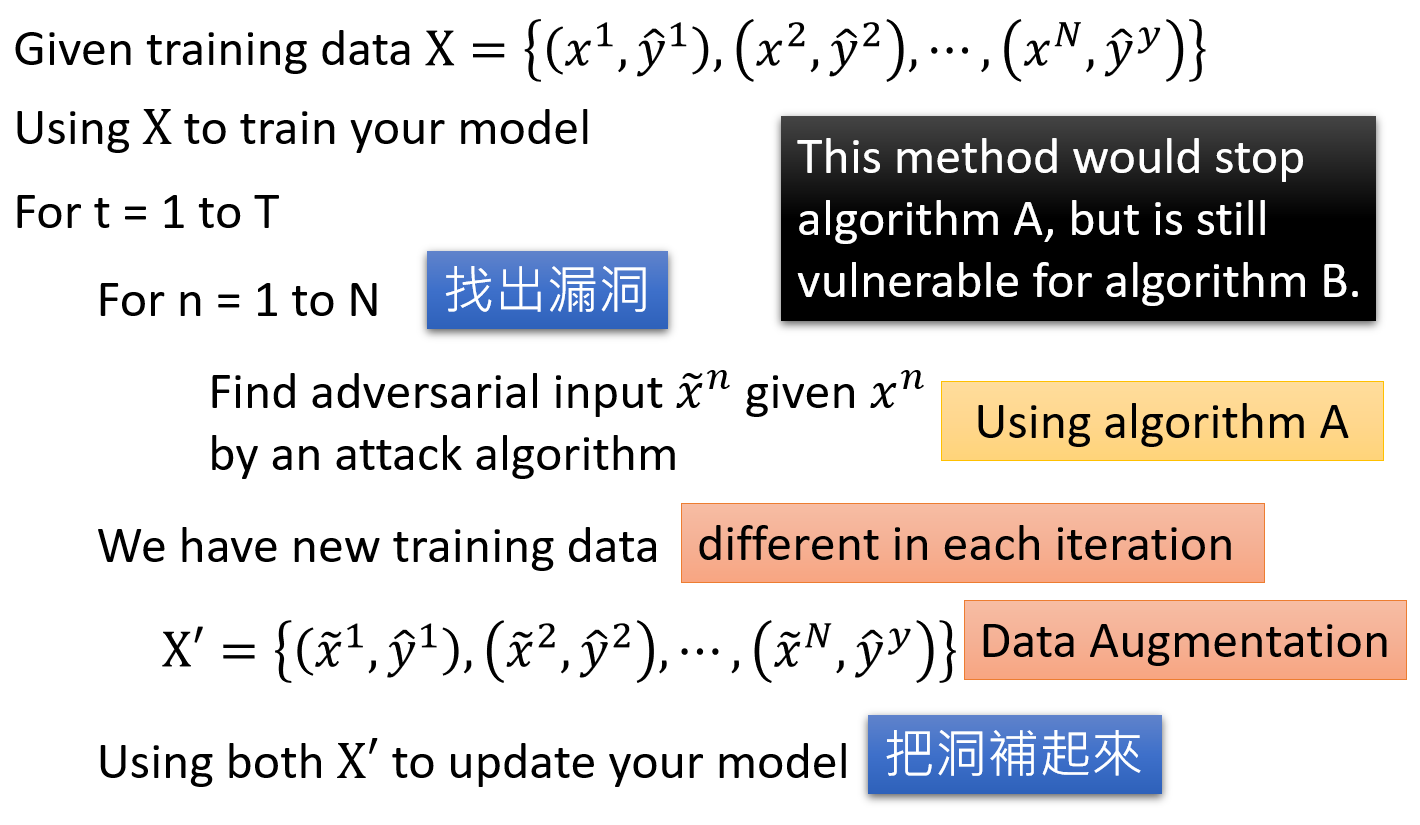## Next Step

Adversarial Robustness - Theory and Practice